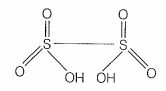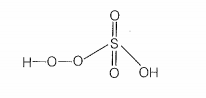Which of the following statements are correct?

a. S — S bond is present in H2S2O6

b. In peroxosulphuric acid (H2SO5) sulphur is in +6 oxidation state

c. Iron powder along with Al2O3 and K2O is used as a catalyst in the preparation of NH3 by Haber's process

d. Change in enthalpy is positive for the preparation of SO3 by catalytic oxidation of SO2

1. (a, b)
2. (b, c)
3. (c, d)
4. (a, d)

Hint: Maximum oxidation state of sulphur is +6

The structure of H2S2O6  is as shown belowIt contains one S—S bond. Hence, first is the correct statement.
(b) In peroxosulphuric acid (H2SO5) sulphur is in + 6 oxidation state.
The structure of H2SO5 islet the oxidation state of S = x:

x - 6 = 0
x = 6

Hence, the second statement is also correct
(c)

During the preparation of ammonia, iron oxide with a small amount of K2O and Al2O3 is used as a catalyst to increase the rate of attainment of equilibrium. Hence the third statement is incorrect.

(d)
Change in enthalpy is negative for the preparation of SO3 by catalytic oxidation of SO2. Hence, fourth statement is wrong.